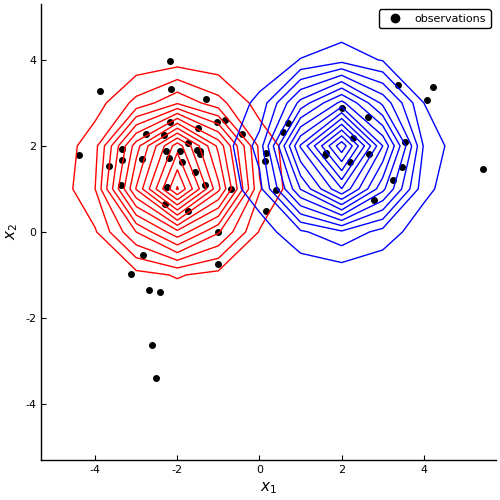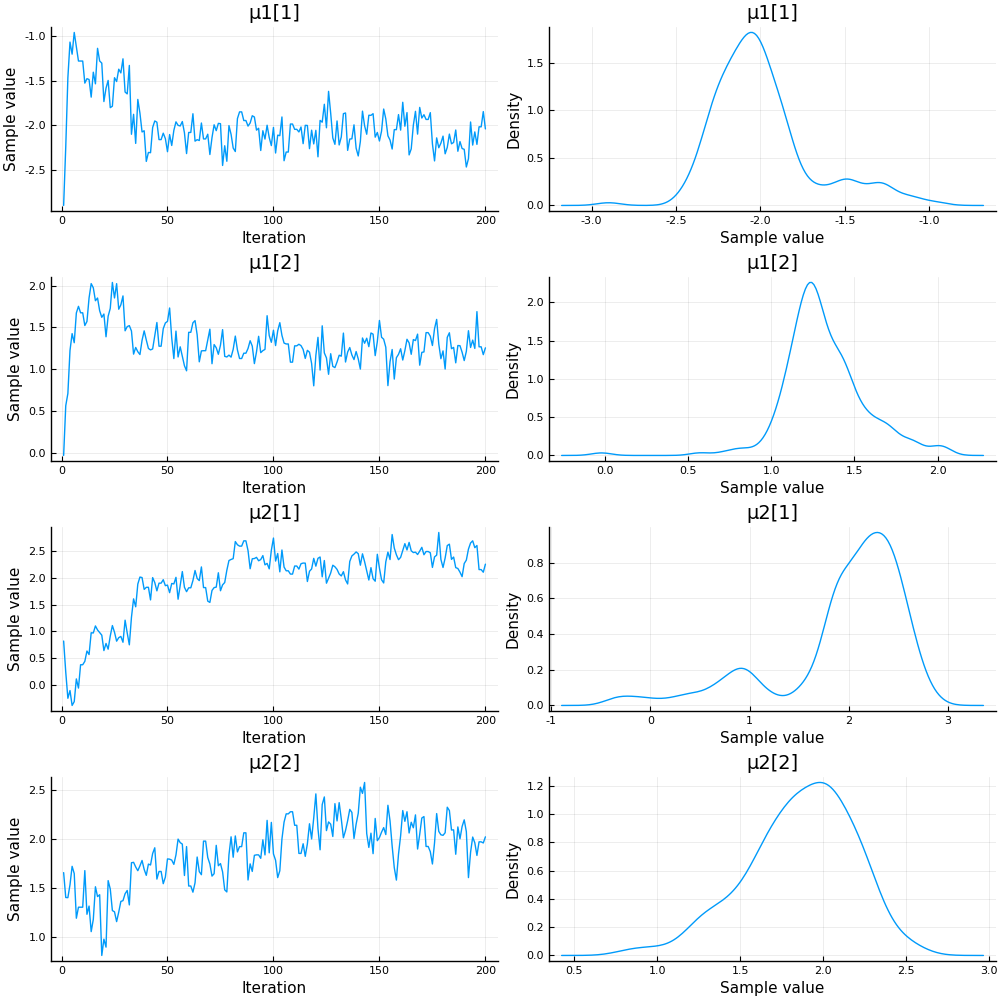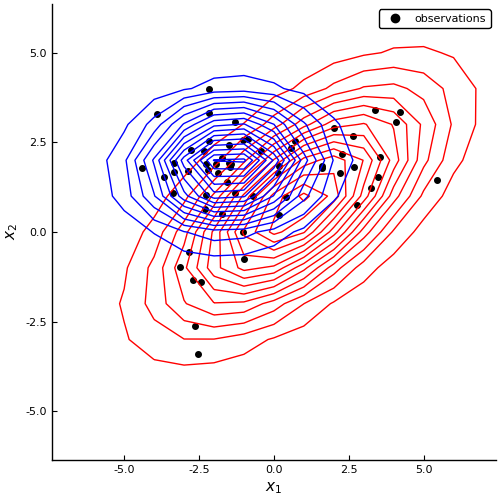# Probabilistic Programming 2: Mixture modeling¶

## Monte Carlo sampling¶

In this notebook, we are exploring a more complex problem set, namely mixture modeling. It will show you how to extend the sampling procedure to the multivariate case.

### Preliminaries¶

• Goal
• Write a Monte Carlo sampling procedure for a 2-dimensional mixture model.
• Materials
• Mandatory
• These lecture notes.
• Optional
In :
# Package managing
using Pkg
Pkg.activate("workspace")
Pkg.instantiate()

using Logging; disable_logging(LogLevel(0))
using Distributions
using StatsPlots
using LaTeXStrings


## Data generation¶

We generate samples from three 2-dimensional Gaussian distributions and mix these. These Gaussians are:

\begin{align*} \mathcal{N}_1(\begin{bmatrix}-2, -2 \end{bmatrix}, \begin{bmatrix}1 & 0 \\0 & 3 \end{bmatrix}) \, , \quad \mathcal{N}_2(\begin{bmatrix}+2, +2 \end{bmatrix}, \begin{bmatrix}3 & 0 \\0 & 1 \end{bmatrix}) \, , \quad \mathcal{N}_3(\begin{bmatrix}-2, +2 \end{bmatrix}, \begin{bmatrix}1 & 0 \\0 & 1 \end{bmatrix}) \, . \end{align*}

We draw $N_1$, $N_2$ and $N_3$ samples from each, respectively.

In :
# Sample size
N = [10, 20, 30]

# Parameters for each cluster
μ1 = [-2. -2.]
Σ1 = [1.0 0.0; 0.0 3.0]
μ2 = [+2. +2.]
Σ2 = [3.0 0.0; 0.0 1.0]
μ3 = [-2. +2.]
Σ3 = [1.0 0.0; 0.0 1.0]

function generate_data(means::Array{Float64,2},
covariances::Array{Float64,3};
num_samples::Array{Int64,1}=)
"Generate data from a 2-dimensional mixture model"

# Extract number of components
K = length(num_samples)

# Extract dimensionality
D = size(covariances)

# Preallocate vectors
X = zeros(sum(num_samples), D)
C = zeros(sum(num_samples),)

# Create sample index
ix = cat(0, cumsum(num_samples), dims=1)

for k = 1:K

# Current indices
ix_k = (ix[k]+1:ix[k+1])

# Sample from cluster component
X[ix_k, :] = rand(MvNormal(means[k,:], covariances[:,:,k]), num_samples[k])'

# Store cluster origin
C[ix_k,] = k*ones(num_samples[k])

end

return X, C
end

# Generate data
observations, cluster = generate_data(cat(μ1, μ2, μ3, dims=1), cat(Σ1, Σ2, Σ3, dims=3), num_samples=N)

# Visualization
scatter(observations[cluster.==1, 1], observations[cluster.== 1, 2], marker=:circle,label="C1")
scatter!(observations[cluster.==2, 1], observations[cluster.== 2, 2], marker=:xcross, label="C2")
scatter!(observations[cluster.==3, 1], observations[cluster.== 3, 2],
marker=:diamond,
label="C3",
xlabel=L"$x_1$",
ylabel=L"$x_2$",
xlim=[-6, 6],
ylim=[-6, 6],
size=(500,500),
grid=false)

Out:

## Model specification¶

Let's quickly recap the $K$-component Gaussian mixture model.

We have a data set of $X$ of $N$ samples by $D$ features. Each component is a Gaussian distribution, $p_k(x \mid \mu_k, \Sigma_k) \triangleq \mathcal{N}(x \mid \mu_k, \Sigma_k)$. In total, we model $N$ samples:

$$p(X \mid z, \mu, \Sigma) = \sum_{i=1}^{N} \sum_{k=1}^{K} p_k(x_i \mid \mu_k, \Sigma_k)^{z_i=k} \, .$$

The variable $z_i$ is the assignment of sample $i$ to class $k$. The probability $p(z_{i} = k)$ is also called the responsibility of component $k$ for the $i$-th sample. All $z_i$ follow a Categorical distribution with mixture weights as parameters:

$$p(z_i) \sim \text{Cat}(\phi) \, ,$$

Being Bayesians, we put priors on all unknown parameters ($\phi, \mu, \Sigma$):

\begin{align} p(\phi) \sim&\ \text{Dir}(a_0) \\ p(\mu) \sim&\ \mathcal{N}(m_0, l_0^{-1}\Sigma) \\ p(\Sigma) \sim&\ \mathcal{W}(W_0, v_0) \end{align} \, .

The distribution for the mixture weights, $p(\phi)$, follows a Dirichlet distribution because it is a vector that should sum to one. The component parameter priors are a Wishart distribution for the covariance matrix and a Gaussian distribution for the mean, conditioned on the sampled covariance matrix. Together, these two priors form a Normal-Inverse-Wishart distribution, $p(\mu, \Sigma)$, which is the conjugate prior for the Gaussian components. The full joint distribution is therefore:

\begin{align} p(X, z, \phi, \mu, \Sigma) =&\ p(X \mid z, \phi, \mu, \Sigma)\ p(z \mid \phi)\ p(\mu, \Sigma)\ p(\phi) \\ =&\ \sum_{i=1}^{N} \sum_{k=1}^{K} \mathcal{N}(x_i \mid \mu_k, \Sigma_k)^{z_i=k}\ \text{Cat}(z_i=k \mid \phi_k)\ \mathcal{NIW}(\mu_k, \Sigma_k \mid m_{0k}, l_{0k}, W_{0k}, v_{0k})\ \text{Dir}(\phi_k \mid a_{0k}) \end{align}

We are interested in the posterior distribution over $z$, which assigns the current samples to clusters via $\arg \max_{k} p(z_i = k)$. For example, if the posterior distribution for $z_i$ is $[0.32\ 0.21\ 0.47]$, then the $\arg \max_k$ is 3 and we say that $x_i$ belongs to component 3. We are also interested in the posterior distributions for the component parameters, because these allow us to assign future samples to the current clusters. The posterior for $z, \phi, \mu, \Sigma$ has the form:

$$p(z, \phi, \mu, \Sigma \mid X) = \frac{p(X, z, \phi, \mu, \Sigma)}{\int p(X, z, \phi, \mu, \Sigma)\ \mathrm{d}X} \, .$$

We cannot evaluate the integral in the denominator, but because it only serves as a normalization factor we ignore it for now. Note that the numerator is the joint distribution. Unfortunately, it doesn't simplify to a known form. However, in mixture models, it is usually possible to perform a conditional factorization into the two distributions: if we fix the parameters $\phi, \mu, \Sigma$ to certain values, we obtain the factor:

$$p(z_i \mid X, \phi, \mu, \Sigma) = \sum_{i=1}^{N} \sum_{k=1}^{K} \rho_k^{z_i = k} \, ,$$

where $\rho_k = \phi_k \mathcal{N}(x_i \mid \mu_k, \Sigma_k)$ for all $k$. This is a Categorical distribution! The only problem is that $\rho$ is not normalized correctly. But we know that it should be correctly normalized, after division by the denominator $\int p(X, z, \phi, \mu, \Sigma) \ \mathrm{d}X$. We can therefore perform the following re-parameterization:

$$r_k = \frac{\rho_k}{\sum_{k} \rho_k} \, .$$

Now, $r_k$ are valid responsibilities for the assignment variable $z$. In other words, $p(z_i \mid X, \phi, \mu, \Sigma) \sim \text{Cat}(r_k)$.

If we fix $z_i$ to certain values, it acts as a component selector. Take for instance $z_i = 2$;

\begin{align} p(\phi_2, \mu_2, \Sigma_2 \mid x_i, z_i) =&\quad \mathcal{N}(x_i \mid \mu_1, \Sigma_1)^{0} \ 0\ \ \ \mathcal{NIW}(\mu_1, \Sigma_1 \mid m_{01}, l_{01}, W_{01}, v_{01}) \ \text{Dir}(\phi_1 \mid a_{01}) \\ &+ \mathcal{N}(x_i \mid \mu_2, \Sigma_2)^{1} \ \phi_2\ \mathcal{NIW}(\mu_2, \Sigma_2 \mid m_{02}, l_{02}, W_{02}, v_{02}) \ \text{Dir}(\phi_2 \mid a_{02}) \\ &+ \mathcal{N}(x_i \mid \mu_3, \Sigma_3)^{0} \ 0\ \ \ \mathcal{NIW}(\mu_3, \Sigma_3 \mid m_{03}, l_{03}, W_{03}, v_{03}) \ \text{Dir}(\phi_3 \mid a_{03}) \\ =& \ \mathcal{N}(x_i \mid \mu_2, \Sigma_2) \ \phi_2\ \mathcal{NIW}(\mu_2, \Sigma_2 \mid m_{02}, l_{02}, W_{02}, v_{02}) \ \text{Dir}(\phi_2 \mid a_{02}) \end{align} \, .

Fixing all $z_i$ produces $p(\phi, \mu, \Sigma \mid X, z)$. This can be further factorized into $p(\phi \mid z) p(\mu, \Sigma \mid X)$:

\begin{align} p(\phi_k \mid z) =&\ \sum_{i=1}^{N} \text{Cat}(z_i \mid \phi_k)\ \text{Dir}(\phi_k \mid a_{0}) \\ p(\mu_k, \Sigma_k \mid X) =&\ \sum_{i=1}^{N} \mathcal{N}(x_i \mid \mu_k, \Sigma_k)\ \mathcal{NIW}(\mu_k, \Sigma_k \mid m_{0k}, l_{0k}, W_{0k}, v_{0k}) \end{align}

which are Dirichlet and a Normal-Inverse-Wishart distributions. So, to summarize:

1. Fix $\phi, \mu, \Sigma$ and compute $p(z \mid X, \phi, \mu, \Sigma)$
2. Fix $z$ and compute both $p(\phi \mid z)$ and $p(\mu, \Sigma \mid X)$.

You will recognize this as being similar to Expectation-Maximization. The difference lies in that we obtain posterior distributions for $\phi, \mu, \Sigma$ instead of point estimates.

Since the posterior factorizes into marginal distributions, we can apply Gibbs sampling to obtain estimates of the posterior parameters.

In :
using Turing
using MCMCChains
include("../scripts/pp-2.jl");


Let's start simple and slowly increase the complexity of the model.

### Model 1: means only¶

We will assume K=2 classes, equal class proportions, $\phi = [0.5 \ 0.5]$, and spherical covariance matrices, $\Sigma_k = \mathcal{I}$ for all $k$. Using these assumptions, we estimate the means $\mu$ and compute the posterior of $z$.

In :
@model GaussianMixtureModel(X, m1, m2) = begin
"2-dimensional Gaussian mixture model with spherical covariances"

# Data shape
N, D = size(X)

# Draw the mean for cluster 1 [2x1 vector]
μ1 ~ MvNormal(m1, 10*[1. 0.; 0. 1.])

# Draw the mean for cluster 2 [2x1 vector]
μ2 ~ MvNormal(m2, 10*[1. 0; 0. 1.])

# Concatenate means [2x2 matrix]
μ = [μ1 μ2]

# Probability of belonging to each cluster [2x1 vector]
ϕ = [0.5, 0.5]

# Preallocate assignment vector [Nx1 vector]
z = Vector{Int}(undef, N)

# Loop over observations
for i in 1:N

# Draw assignment for i-th sample from a Categorical distribution parameterized by ϕ
z[i] ~ Categorical(ϕ)

# Data point x[i] follows a normal distribution, with mean indexed by the assignment variable z[i]
X[i,:] ~ MvNormal(μ[:, z[i]], [1. 0; 0. 1.])
end

# Return the assignments
return z
end

# Set priors
m1 = [-2, -2]
m2 = [+2, +2]

# Create an instance of a model, using the observations
model1 = GaussianMixtureModel(observations, m1, m2);


Well construct a Gibbs sampler, partly sampling the cluster assignment $z$ and partly the means.

In :
# Length of chain
len_chain = 200

# Construct Gibbs sampler
sampler1 = Gibbs(PG(20, :z), HMC(0.1, 2, :μ1, :μ2))

# Start sampling
chain1 = sample(model1, sampler1, len_chain);

Progress: 100%|█████████████████████████████████████████| Time: 0:01:18

In :
# Extract the means over the MCMC chains
μ1_hat = mean_chain(chain1, :μ1)
μ2_hat = mean_chain(chain1, :μ2)

# Plot data and overlay estimated posterior probabilities
plot_posteriors(observations, μ1_hat, μ2_hat, x1=range(-5, stop=5), x2=range(-5, stop=5))

Out:Let's take a closer look at the chain.

In :
describe(chain1[[:μ1, :μ2]])

Out:
2-element Array{ChainDataFrame,1}

Summary Statistics
. Omitted printing of 1 columns
│ Row │ parameters │ mean     │ std      │ naive_se  │ mcse      │ ess     │
│     │ Symbol     │ Float64  │ Float64  │ Float64   │ Float64   │ Any     │
├─────┼────────────┼──────────┼──────────┼───────────┼───────────┼─────────┤
│ 1   │ μ1      │ -1.98805 │ 0.300151 │ 0.0212239 │ 0.0936805 │ 7.09726 │
│ 2   │ μ1      │ 1.31618  │ 0.251094 │ 0.017755  │ 0.0690124 │ 10.9588 │
│ 3   │ μ2      │ 1.94179  │ 0.663452 │ 0.0469132 │ 0.358442  │ 3.84963 │
│ 4   │ μ2      │ 1.86004  │ 0.324754 │ 0.0229636 │ 0.219076  │ 2.43755 │

Quantiles

│ Row │ parameters │ 2.5%     │ 25.0%    │ 50.0%    │ 75.0%    │ 97.5%    │
│     │ Symbol     │ Float64  │ Float64  │ Float64  │ Float64  │ Float64  │
├─────┼────────────┼──────────┼──────────┼──────────┼──────────┼──────────┤
│ 1   │ μ1      │ -2.39936 │ -2.17841 │ -2.04652 │ -1.87881 │ -1.25273 │
│ 2   │ μ1      │ 0.883976 │ 1.18098  │ 1.27805  │ 1.43876  │ 1.85292  │
│ 3   │ μ2      │ 0.107808 │ 1.82129  │ 2.13389  │ 2.37234  │ 2.69004  │
│ 4   │ μ2      │ 1.17884  │ 1.66775  │ 1.89319  │ 2.08518  │ 2.37165  │

In :
plot(chain1[[:μ1, :μ2]])

Out:### Model 2: means and covariances¶

We modeled the data with spherical Gaussians. But the data doesn't really look spherical; the cluster plot showed that quite a few samples are not covered by the two Gaussians. This suggests that we should estimate the covariance matrices as well.

A variance parameter is a strictly positive number and is captured with a Gamma distrbution. A covariance matrix is a positive-definite matrix and can be captured with a Wishart distribution. The Wishart distribution is the natural multivariate extension of the Gamma distribution:

$$\mathcal{W}_p(C \mid V, n) = \frac{1}{2^{np/2} \ |V|^{n/2} \ \Gamma_p(n/2)} |C|^{(n-p-1)/2} \exp(-\text{tr}(V^{-1}C)/2)$$

where $p$ is the dimensionality of the matrix-variate, $n$ is the degrees of freedom and $V$ is a scale matrix. $\Gamma_p$ is a multivariate gamma function (continuous version of the factorial function).

It is relatively straightforward to add two Wishart priors to the model:

In :
@model GaussianMixtureModel(X, m1, m2, S1, S2) = begin
"2-dimensional Gaussian mixture model with free covariances"

# Data shape
N, D = size(X)

# Draw a covariance matrix for each cluster [2x2 matrix]
Σ1 ~ Wishart(2, S1)
Σ2 ~ Wishart(2, S2)

# Draw means for each cluster [2x1 vector]
μ1 ~ MvNormal(m1, Σ1)
μ2 ~ MvNormal(m2, Σ2)

# Concatenate parameters to allow for indexing
μ = [μ1 μ2]
Σ = cat(Σ1, Σ2, dims=3)

# Probability of belonging to each cluster [2x1 vector]
ϕ = [0.5, 0.5]

# Preallocate assignment vector [Nx1 vector]
z = Vector{Int}(undef, N)

# Loop over observations
for i in 1:N

# Draw assignment for i-th sample from a Categorical distribution parameterized by ϕ
z[i] ~ Categorical(ϕ)

# Data point x[i] follows a normal distribution, with mean and covariance indexed by z[i]
X[i,:] ~ MvNormal(μ[:, z[i]], Σ[:,:, z[i]])
end

# Return the assignments
return z
end

# Set prior means
m1 = [-1, -1]
m2 = [+1, +1]

# Set prior covariances
S1 = [1. 0.;0. 1.]
S2 = [1. 0.;0. 1.]

# Create an instance of a model, using the observations
model2 = GaussianMixtureModel(observations, m1, m2, S1, S2);


We'll have to extend the sampler as well. For now, we'll include $\Sigma1$ and $\Sigma2$ in the Hamiltonian MC procedure.

In :
# Length of chain
len_chain = 100

# Construct Gibbs sampler
sampler2 = Gibbs(PG(20, :z), HMC(0.1, 2, :μ1, :μ2, :Σ1, :Σ2))

# Start sampling
chain2 = sample(model2, sampler2, len_chain);

Progress: 100%|█████████████████████████████████████████| Time: 0:03:18


Let's extract the sample averages for the means and covariances of the clusters.

In :
# Extract the means over the MCMC chains
μ1_hat = mean_chain(chain2, :μ1)
μ2_hat = mean_chain(chain2, :μ2)
Σ1_hat = reshape(mean_chain(chain2, :Σ1), (2,2))
Σ2_hat = reshape(mean_chain(chain2, :Σ2), (2,2))

# Plot data and overlay estimated posterior probabilities
plot_posteriors(observations, μ1_hat, μ2_hat, Σ1=Σ1_hat, Σ2=Σ2_hat, x1=range(-7, stop=7), x2=range(-6, stop=6))

Out:Notice that we've improved coverage of all the points, but the fit is still not perfect.

In :
plot(chain2[[:μ1, :Σ1, :μ2, :Σ2]])

Out: